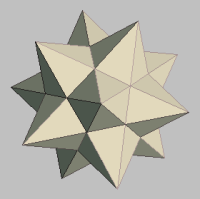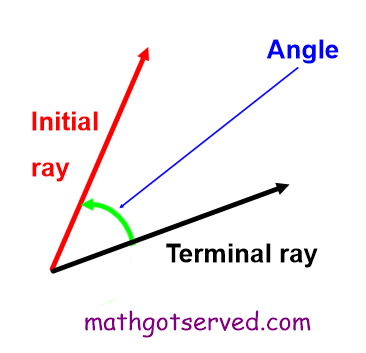MATHGOTSERVEDMATH DICTIONARY
AAngle
Definition: An angle is the inclination or turn from one ray (initial) to another ray (terminal) relative to a common point.
Domain and Range:
Definition: Graphically the domain can be described as the horizontal span of the graph of a function when it is projected on the x-axis. The domain can be thought of as the width of the graph of a function. The range on the other hand is the vertical span of the graph of a function when it is projected on the y-axis. It can be thought of as the height of a function.

F
Function
Definition: A function from a set A to a set B is a relation that assigns to each element x in the set A exactly one element y in set B. The set A is the domain and the set B is the range.

S
Slope
The slope of a line is the number of units that a line rises or drops vertically for each unit of horizontal change heading in the (right direction). Note that the rise of a slope has a sign either negative(down) or positive (up) but the run is always to the right.